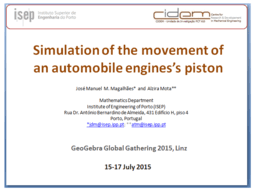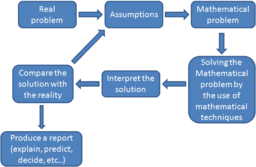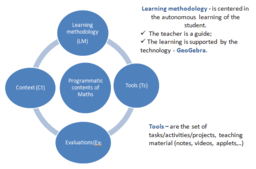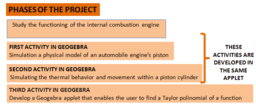# Simulation of the movement of an automobile engine's piston

One of the great challenges of mathematics teachers is to keep students motivated to the study of math. The abstraction and the lack of applicability of the subjects taught has been the major cause of dropout in this discipline. Nowadays, the technologies can be used to support teaching, making maths a tangible science. In this work, we present two activities inserted into a project proposed to the students of the degree in Mechanical Engineering of the Superior Institute of Engineering of Porto – Polytechnic Institute of Porto (ISEP), within the framework of Math discipline. These activities aim the contact with parametric and trigonometric functions, thermodynamic concepts and series of functions applied to the physical model of a piston of an automobile engine. In one activity, the students have developed an applet in Geogebra to simulate and consequently, explore the operation of a cylindrical piston of an automobile engine. In the other, the students have created an applet to obtain a polynomial approximation of a given function. The implementation of these tasks led to the consolidation of mathematical concepts involved in a context of simulation and mathematical modeling.
•### Presentation

•### Theoretical Framework

•### Learning contents of maths by projects

•### Project: Simulation of the movement of an automobile engine's piston

••